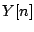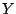Next: Filters Up: Time shifts and delays Previous: Pitch shifter   Contents   Index

# Exercises

1. A complex number has magnitude one and argument. What are its real and imaginary parts?

2. A complex number has magnitude one and real part. What is its imaginary part? (There are two possible values.)

3. What delay time would you give a comb filter so that its first frequency response peak is at 440 Hertz? If the sample rate is 44100, what frequency would correspond to the nearest integer delay?

4. Suppose you made a variation on the non-recirculating comb filter so that the delayed signal was subtracted from the original instead of adding. What would the new frequency response be?

5. If you want to make a 6-Hertz vibrato with a sinusoidally varying delay line, and if you want the vibrato to change the frequency by 5%, how big a delay variation would you need? How would this change if the same depth of vibrato was desired at 12 Hertz?

6. A complex sinusoidhas frequency 11025 Hertz, amplitude 50 and initial phase 135 degrees. Another one,, has the same frequency, but amplitude 20 and initial phase 45 degrees. What are the amplitude and initial phase of the sum ofand?

7. What are the frequency, initial phase, and amplitude of the signal obtained when(above) is delayed 4 samples?

8. Show that the frequency response of a recirculating comb filter with delay timeand feedback gain, as a function of angular frequency, is equal to:Next: Filters Up: Time shifts and delays Previous: Pitch shifter   Contents   Index
Miller Puckette 2006-12-30